# Series

What is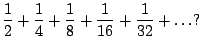What is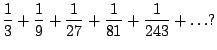What is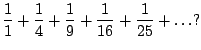Consider the following sequence of partial sums: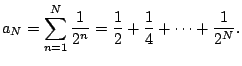Can we compute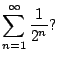These partial sums look as follows:It looks very likely that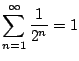, if it makes any sense. But does it?

In a moment we will define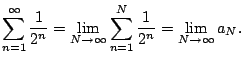A little later we will show that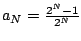, hence indeed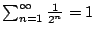.

Definition 6.2.1 (Sum of series)   If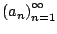is a sequence, then the sum of the series is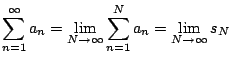provided the limit exists. Otherwise we say that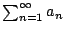diverges.

Example 6.2.2 (Geometric series)   Consider the geometric series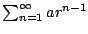for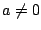. Then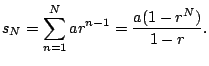To see this, multiply both sides by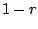and notice that all the terms in the middle cancel out. For what values of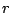does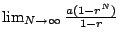converge? If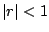, then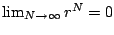and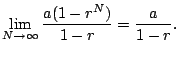If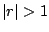, then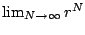diverges, sodiverges. If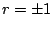, it's clear sincethat the series also diverges (since the partial sums are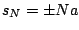).

For example, if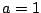and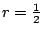, we get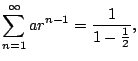as claimed earlier.

William Stein 2006-03-15